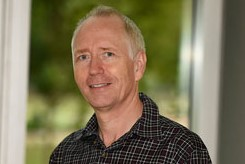COSAM » Events » 2021 » November » DMS Applied Mathematics Seminar

 DMS Applied Mathematics Seminar Time: Nov 05, 2021 (03:00 PM) Location: 228 Parker Hall Details:Speaker: Rudi Weikard, UAB   Title: Rough coefficients in ordinary differential equations   Abstract: We investigate the spectral theory for the system $$Ju’+qu=w(\lambda u+f)$$ of ordinary differential equations where $$J$$ is constant invertible skew-hermitian matrix while $$q$$ is a hermitian and $$w$$ a non-negative matrix whose entries are distributions of order $$0$$. A major obstacle is the fact that, in general, the unique continuation of solutions of the differential equation is not possible. Basic information on distributions will be provided.

Last updated: 11/03/2021